# Basic Geometrical Ideas Class 6 Extra Questions Maths Chapter 4

ncert textbook

## Basic Geometrical Ideas Class 6 Extra Questions Maths Chapter 4

Extra Questions for Class 6 Maths Chapter 4 Basic Geometrical Ideas

### Basic Geometrical Ideas Class 6 Extra Questions Very Short Answer Type

Question 1.
Draw a rough sketch of:
(a) open curve
(b) closed curve
Solution:Question 2.
Draw a rough sketch of closed curve made up of line segments.
Solution:
Required curve is ABCD closed with the line segments$\overline { AB }$,$\overline { BC }$,$\overline { CD }$and$\overline { DA }$.Question 3.
Draw two different angles having common point and a common arm.
Solution:
∠AOB and ∠COB are two different angles with common point O and common arm$\overrightarrow { OB }$.
IMG

Question 4.
Identify the points which are:
(i) in the interior
(ii) in the exterior
(iii) on the closed curve in the given figure.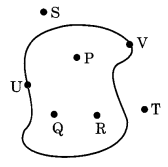Solution:
(i) Points P, Q and R are in the interior of the closed curve.
(ii) points S and T are in the exterior of the closed curve.
(iii) U and V are on the closed curve.

Question 5.
Identify the following in the given figure:
(a) Sector
(b) Chord
(c) Diameter
(d) Segment.Solution:
(a) OPR (shaded) is the sector of the circle.
(b)$\overline { MN }$is the chord.
(c)$\overline { PQ }$is the diameter.
(d) MXN (shaded) is the segment.

Question 6.
In the given figure, name all the possible triangles.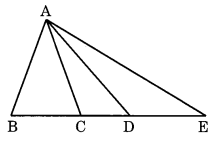Solution:
Possible triangles are:
(i) ∆ABC
(ii) ∆ABD
(iii) ∆ABE
(iv) ∆ACD
(a) ∆ACE

Question 7.
Name all the angles in the given figure.
Solution:
In the given figure, the names of all the angles are: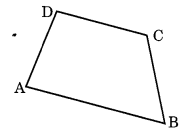(i) ∠ABC
(ii) ∠BCD
(iii) ∠CDA
(iv) ∠DAB

Question 8.
In the given figure, name all the line segments: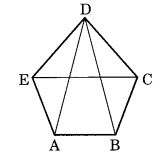Solution:
In the given figure, the name of the line segments are:$\overline { AB}$,$\overline { BC }$,$\overline { CD }$,$\overline { DE }$,$\overline { EA }$,$\overline { DA }$,$\overline { DB }$and$\overline { EC }$.

### Basic Geometrical Ideas Class 6 Extra Questions Short Answer Type

Question 9.
Using the given figure, name the following: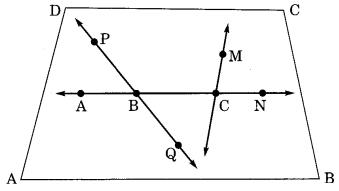(a) Line containing point M.
(b) Line passing through four points.
(c) Line passing through three points.
(d) Two pairs of intersecting lines.
Solution:
(a)$\overleftrightarrow { MC }$is the line containing the point M.
(b)$\overleftrightarrow { AN }$is the line passing through four points A, B, C and N.
(c)$\overleftrightarrow { PQ }$is the line passing through three points P, B and Q.
(d) Pairs for intersecting lines are
(i)$\overleftrightarrow { AN }$and$\overleftrightarrow { PQ }$
(ii)$\overleftrightarrow { AN }$and$\overleftrightarrow { MC }$

Question 10.
On the given line, some points are given, write down the names of all segments.Solution:
Segments are:$\overline { PQ }$,$\overline { PR }$,$\overline { PS }$,$\overline { PT }$,$\overline { QR }$,$\overline { QS }$,$\overline { QT }$,$\overline { RS }$,$\overline { RT }$,$\overline { ST }$.

Question 11.
How many lines can pass through
(i) one given point?
(ii) two given points?
(iii) three non-collinear points
Solution:
(i) Infinite number of lines can be passed through one given point.
(ii) Only one line can pass through two given points.
(iii) Three lines can pass through three non- collinear points.Question 12.
Look at the given figure and answer the following: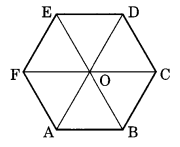(a) Name the sides of the polygon ABCDEF.
(b) Name any two pairs of adjacent sides. .
(c) Name all the segments which intersect each other at one point.
(d) Name all the diagonals of the given polygon.
Solution:
(a) The sides of the polygon are:$\overline { AB }$,$\overline { BC }$,$\overline { CD }$,$\overline { DE }$,$\overline { EF }$and$\overline { FA }$.
(b)$\overline { AB }$and$\overline { BC }$,$\overline { BC }$and$\overline { CD }$are the pairs of adjacent sides.
(c)$\overline { AD }$,$\overline { BE }$and$\overline { CF }$intersect each other at O.
(d) Name of the diagonals are:$\overline { AD }$,$\overline { BE }$and$\overline { CF }$.

Question 13.
Fill in the blanks.
(a) ……… is the largest chord of a circle.
(b) ……… divides the circle into two equal semi circles.
(c) Rectangle is a ……… curve.
(d) Triangle has ……… angles and three ……… .
(e) Only one line can be drawn through ……… points.
(f) ……… number of lines can pass through a given point.
(g) A closed figure made up of entirely of line segments is called a ……… .
(h) A curve which does not cross itself is called a ……… curve.
(i) The length of boundary of a circle is called its ……… .
Solution:
(a) Diameter
(b) Diameter
(c) Closed
(d) three, sides
(e) two
(f) Infinite
(g) polygon
(h) simple
(i) circumference

### Basic Geometrical Ideas Class 6 Extra Questions Long Answer Type

Question 14.
Draw the medians of a AABC and answer the following:
(а) Name the three medians.
(b) Do the medians intersect each other at the same point?
(c) What is that point called?
(d) Can this point be outside the triangle?Solution:
(a) Names of the medians are$\overline { AD }$,$\overline { BE }$and$\overline { CF }$.
(b) Yes, the medians intersect each other at the same point G.
(c) The point of intersection of the medians of a triangle is called ‘Centroid’.
(d) No, this point cannot be out of the triangle.

### Basic Geometrical Ideas Class 6 Extra Questions Multiple Choice Type

Question 15.
Least number of line segments required to make a polygon is
(a) 1
(b) 2
(c) 3
(c) 4
Solution:
Correct option is (c).

Question 16.
How many lines can be drawn through given two points?
(a) Only one
(b) 2
(c) 4
(d) Countless
Solution:
Correct option is (a).

Question 17.
How many vertices are there in a triangle?
(a) 1
(b) 2
(c) 3
(c) 4
Solution:
Correct option is (c).

Question 18.
Say ‘true’ or ‘False’.
(а) Two diameters of a circle will necessarily intersect.
(b) The centre of a circle always lies in the interior.
(c) The diameter is half of the radius of a circle.
(d) Longer chord is nearer to the centre of the circle.
Solution:
(a) True
(b) True
(c) False
(d) True

### Basic Geometrical Ideas Class 6 Extra Questions Higher Order Thinking Skills (HOTS)

Question 19.
Draw an equilateral ∆ABC of any size. Draw AD as its median and an altitude AM.
(i) Does AD coincide with AM?
(ii) Name the point on the median which divides it in the ratio 1:2.
(iv) Are D and M the same points?
Solution:
(i) Yes, AD coincides with AM.(ii) The point on the median which divides it in the ratio 1 : 2 is called centroid of the triangle.
(iv) Yes, D and M are the same points.

Question 20.
In the given figure, l, m and n are three parallel lines, x andy intersect these lines.
(i) Name the points lying on the line x.
(ii) Name the points lying on the liney.
(iii) Name the points inside the quadrilateral ABED.
(iv) Name the points outside the quadrilaterals ABED and BCFE.
(v) Name the lines passing through three points.
Solution:
(i) A, B and C lie on the line x.
(ii) D, E and F lie on the liney.
(iii) Q is the point inside ▢ABED(iv) Points R and S are outside the quadrilaterals ABED and BCFE.
(v) Lines x andy pass through the three points A, B, C and D, E, F respectively.